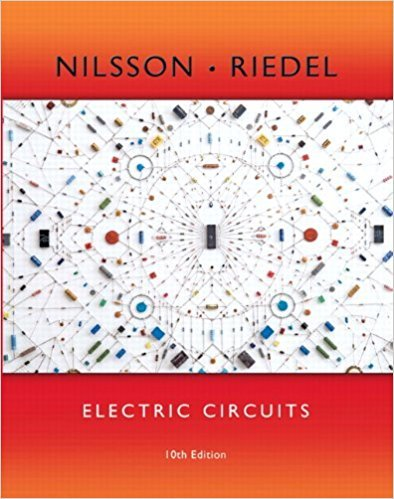×
×

# Solutions for Chapter 13.5: The Transfer Function in Partial Fraction Expansions## Full solutions for Electric Circuits | 10th Edition

ISBN: 9780133760033Solutions for Chapter 13.5: The Transfer Function in Partial Fraction Expansions

Solutions for Chapter 13.5
4 5 0 361 Reviews
28
0
##### ISBN: 9780133760033

Since 2 problems in chapter 13.5: The Transfer Function in Partial Fraction Expansions have been answered, more than 9573 students have viewed full step-by-step solutions from this chapter. This expansive textbook survival guide covers the following chapters and their solutions. Chapter 13.5: The Transfer Function in Partial Fraction Expansions includes 2 full step-by-step solutions. This textbook survival guide was created for the textbook: Electric Circuits, edition: 10. Electric Circuits was written by and is associated to the ISBN: 9780133760033.

Key Engineering and Tech Terms and definitions covered in this textbook
×